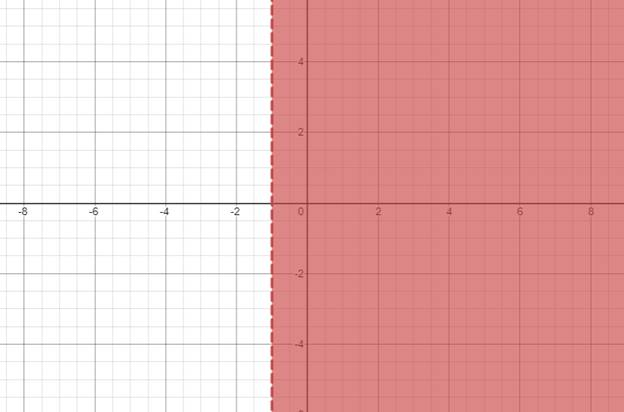# The solution of given nonlinear inequality equation and plot a graph for it.### Precalculus: Mathematics for Calcu...

6th Edition
Stewart + 5 others
Publisher: Cengage Learning
ISBN: 9780840068071### Precalculus: Mathematics for Calcu...

6th Edition
Stewart + 5 others
Publisher: Cengage Learning
ISBN: 9780840068071

#### Solutions

Chapter 1.7, Problem 52E
To determine

## The solution of given nonlinear inequality equation and plot a graph for it.

Expert Solution

x<1,x>3 and x>3

### Explanation of Solution

Given:

Non-linear inequality equation

(x+3)2(x+1)>0

Calculation,

Non-linear inequality equation,

(x+3)2(x+1)>0(x+3)(x+3)(x+1)>0Now,x+1>0x<1andx+3>0x>3andx+3>0x>3

Now, graph of (x+3)2(x+1)>0Conclusion:

Hence, the values of x is x<1,x>3 and x>3 .

### Have a homework question?

Subscribe to bartleby learn! Ask subject matter experts 30 homework questions each month. Plus, you’ll have access to millions of step-by-step textbook answers!